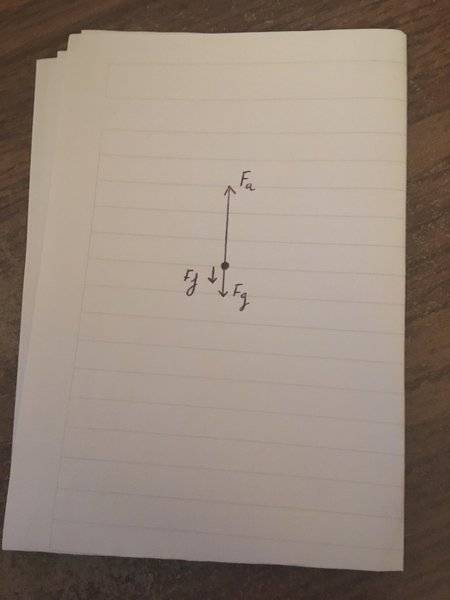# A question about Newton's laws & free body diagrams....

thebosonbreakerHere I have drawn the free-body diagram for a rock (but could really be any object) which is being pulled upwards.
Fa represents the applied force (let's say...by a rope)
Fg represents gravity/weight of the object
Ff represents friciton, i.e. the small amount of air resistance

But here's my question.
By Newton's 3rd law of motion if a force is exerted on an object then the object will exert a force which is equal in magnitude and opposite in direction. Essentially, all forces act in pairs.
Still assuming that the object is attached to a rope and is being pulled up by it, then the rope is clearly exerting a force on the rock (causing it to accelerate upwards). But what is the reaction force to this? Is there a force being exterted on the rope by the rock but it's insignificant and thus not included in the diagram?

If anybody could clear up this element of confusion of mine I'd be really grateful!

#### Attachments

•Dale

Mentor
View attachment 217714 Here I have drawn the free-body diagram for a rock ...

But what is the reaction force to this? Is there a force being exterted on the rope by the rock but it's insignificant and thus not included in the diagram?.
The force on the rope is not included on the free body diagram because the free body diagram is for the rock. Only forces acting on the rock are shown on the rock’s free body diagram.

If you also made a free body diagram for the rope then you would include the reaction force on the rope’s diagram. It would be “equal and opposite” the force on the rock, per Newton’s third law.

•sophiecentaur and thebosonbreaker
Staff Emeritus
Homework Helper
Gold Member
This is a common misconception. The point is that the third law partner of a force acts on a different system. In this case, you are drawing the FBD of the rock and therefore you include all external forces on the rock. For example, the force on the rock from the rope. If you in addition drew the FBD for the rope, then that FBD would include the force from the stone on the rope, ie, the third law partner of the force from the rope on the rock.

The point is that by definition, the third law partners of whatever forces you draw in a FBD will be not be acting on the system you are drawing the FBD for.

•sophiecentaur, thebosonbreaker and Dale
Your diagram shows the forces on the rock. That's what you need to evaluate what the rock does. You could draw a diagram for the rope, and figure out what the rope does. The important thing to remember is each member of a force pair acts on different objects. one Acts on the rock, the other acts on the rope.

Oro beat me to it

& Dale beat Oro!

•Dale, sophiecentaur and thebosonbreaker
Staff Emeritus
Homework Helper
Gold Member
This must be some sort of PF record. Three essentially equivalent answers given within a 2 minute window 6-8 minutes after the OP ...

•Dale, thebosonbreaker, anorlunda and 1 other person
thebosonbreaker
This must be some sort of PF record. Three essentially equivalent answers given within a 2 minute window 6-8 minutes after the OP ...
Haha, I agree!

thebosonbreaker
•